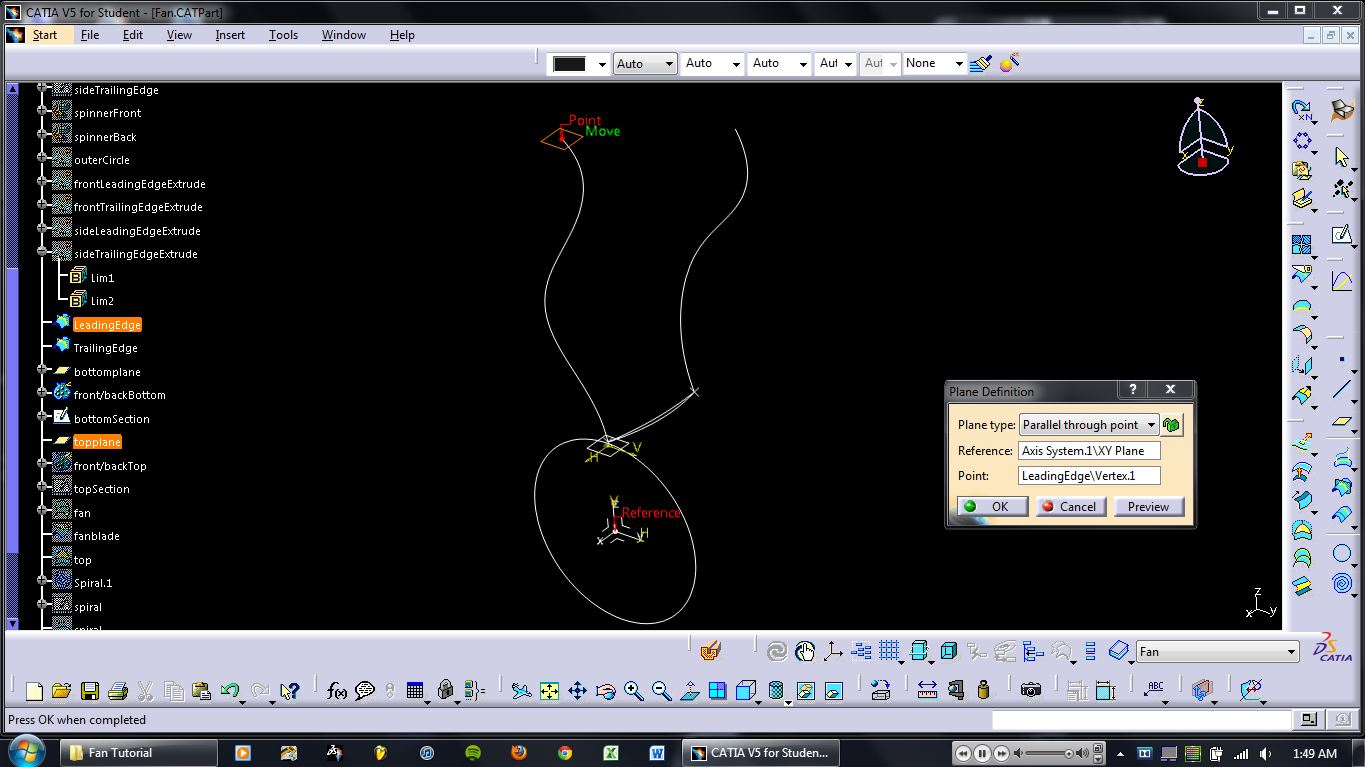# 2nd tutorial on how to model a wide-chord fan for a turbofan engine in CATIA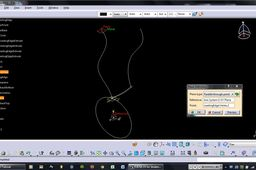Steps 1 thru 15.

1. ### Step 1:

1. Create the circle on the yz-plane. Exit sketcher. Be sure to name your sketches, otherwise it's difficult to keep track of everything.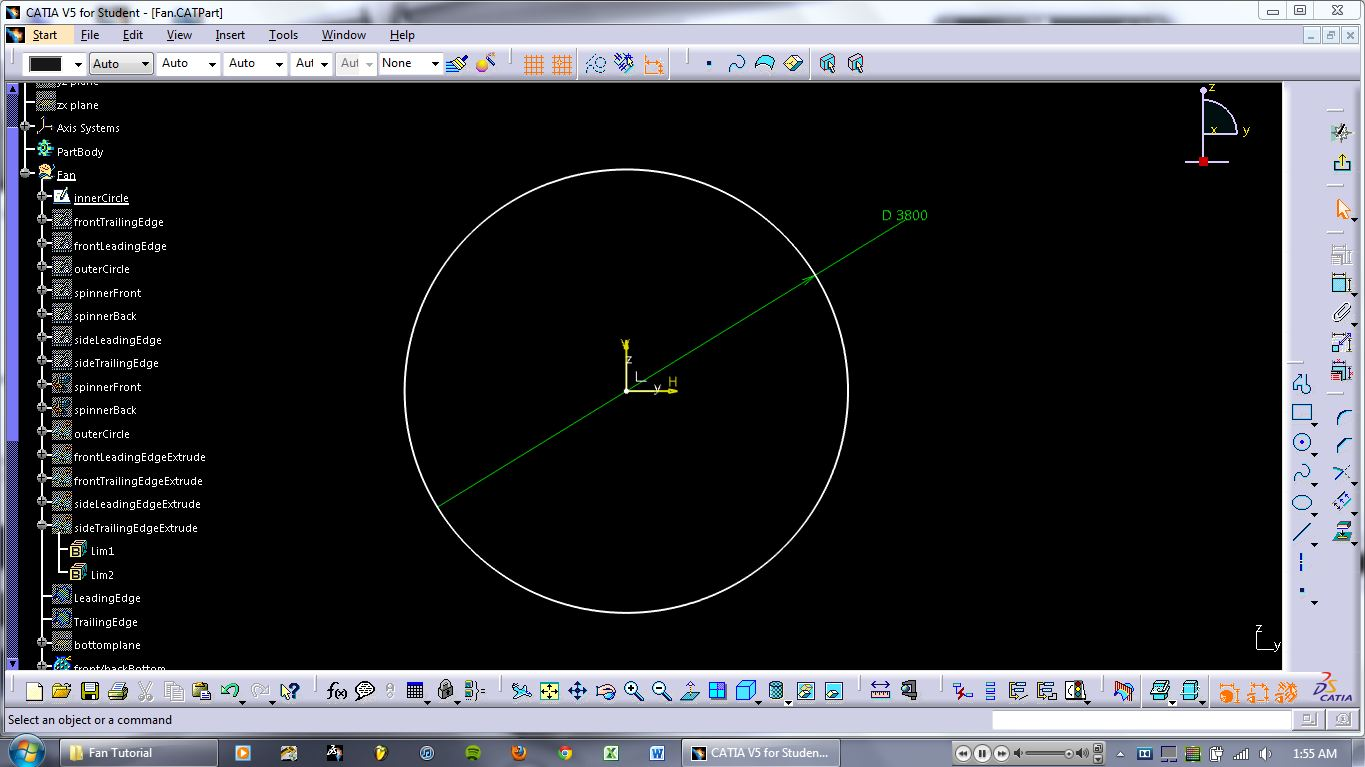2. ### Step 2:

2. Enter sketcher on the yz-plane and create the spline shown. Exit sketcher.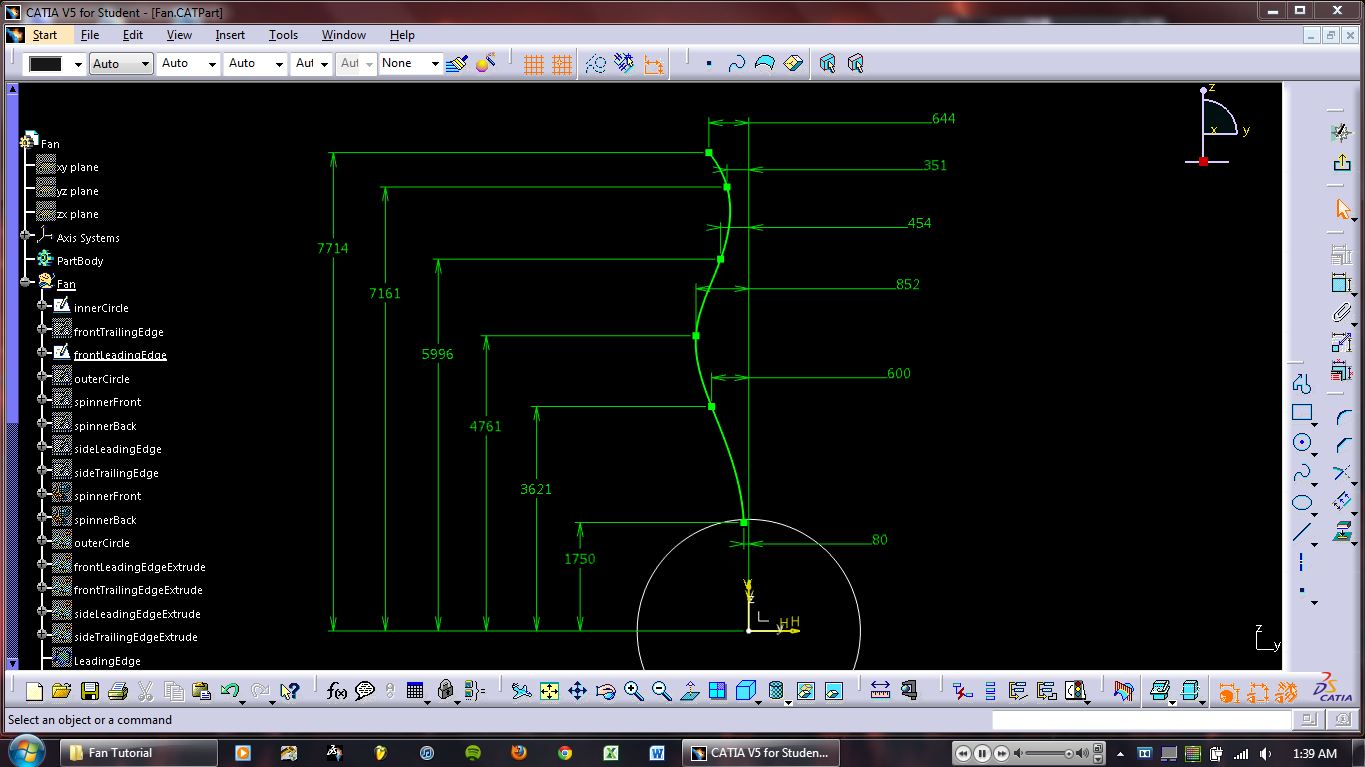3. ### Step 3:

3. Create the following spline on the yz-plane. Exit sketcher.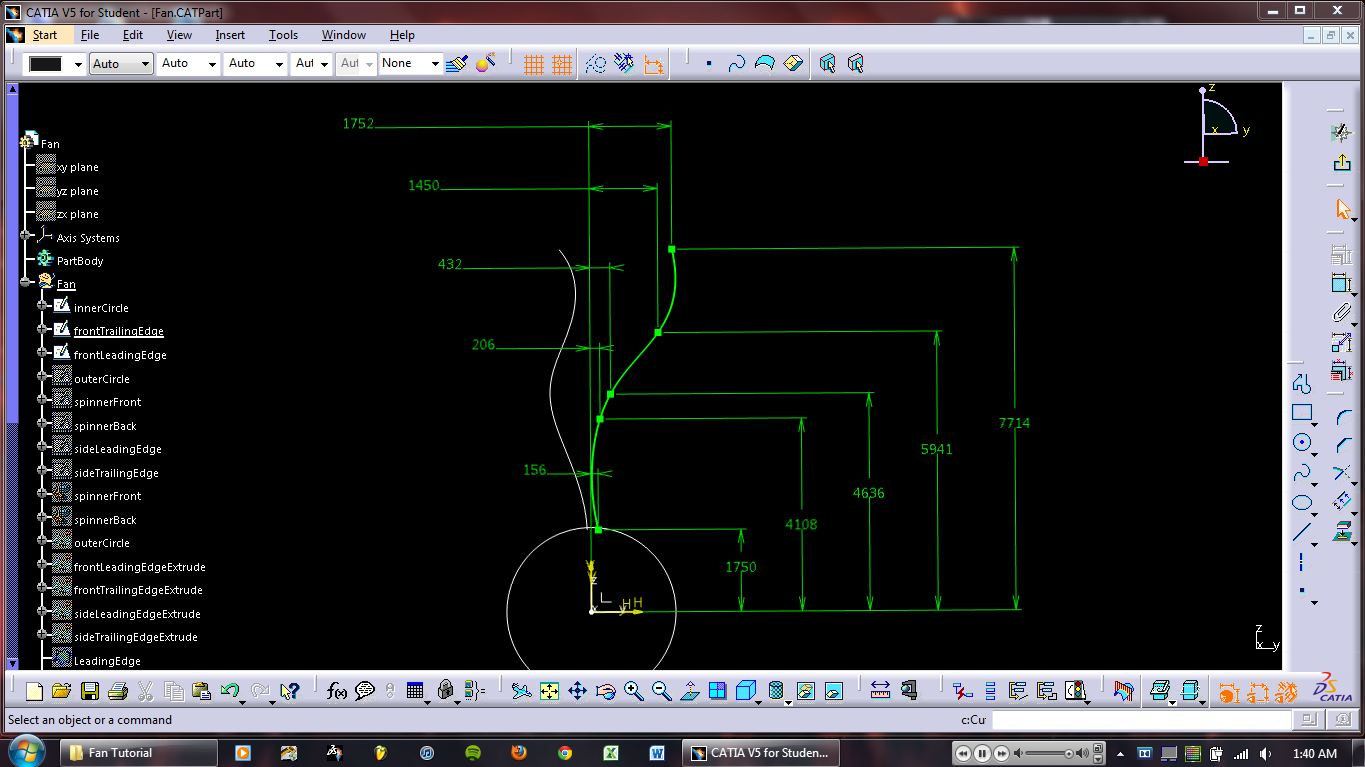4. ### Step 4:

4. Create the following spline on the zx-plane. Exit sketcher.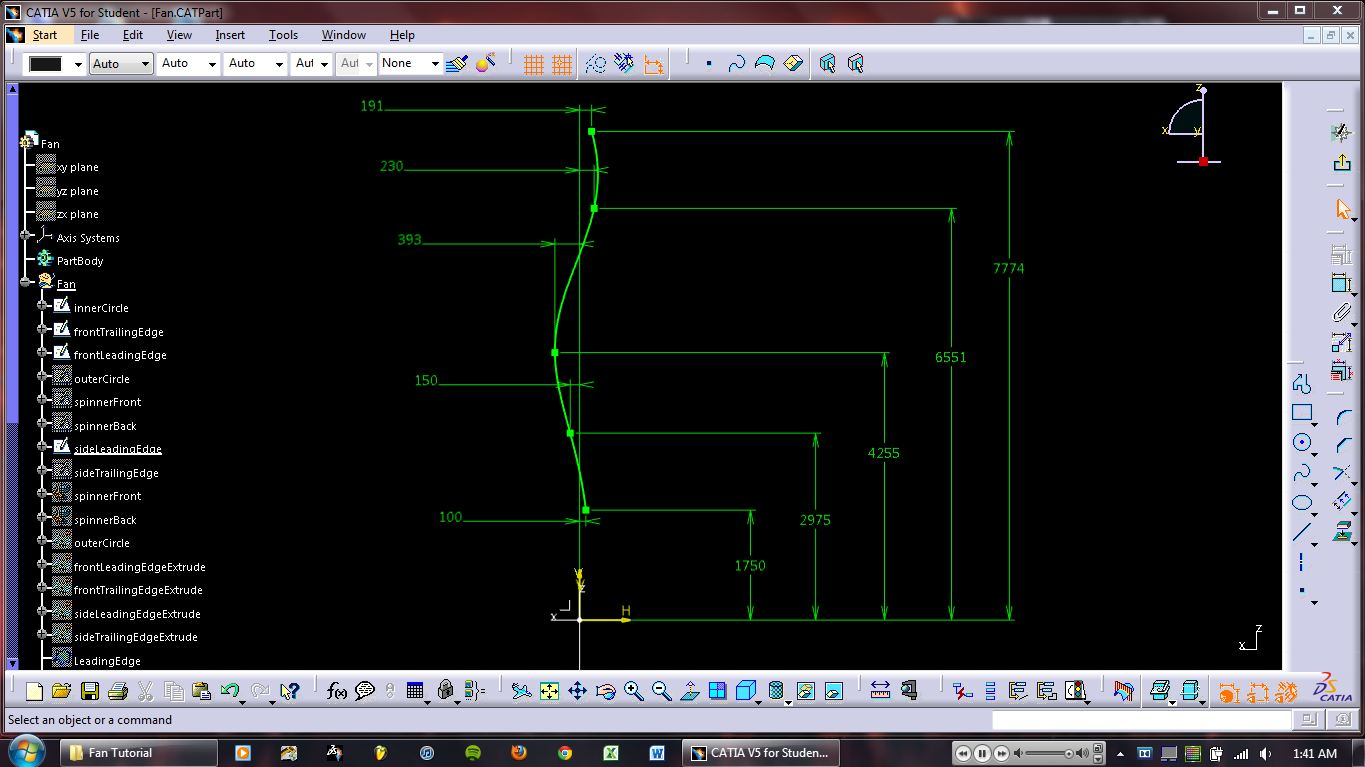5. ### Step 5:

5. Create the following spline on the zx-plane. Exit sketcher.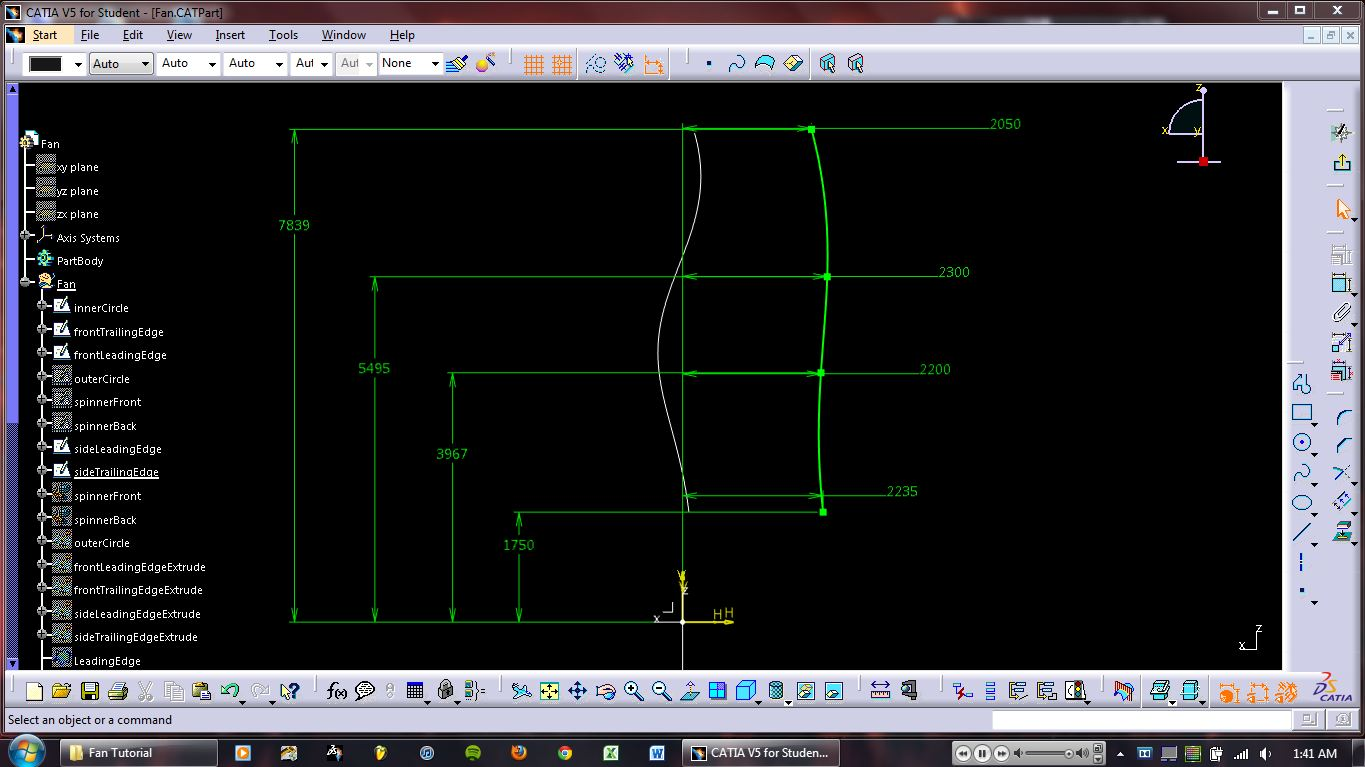6. ### Step 6:

6. Extrude the first spline.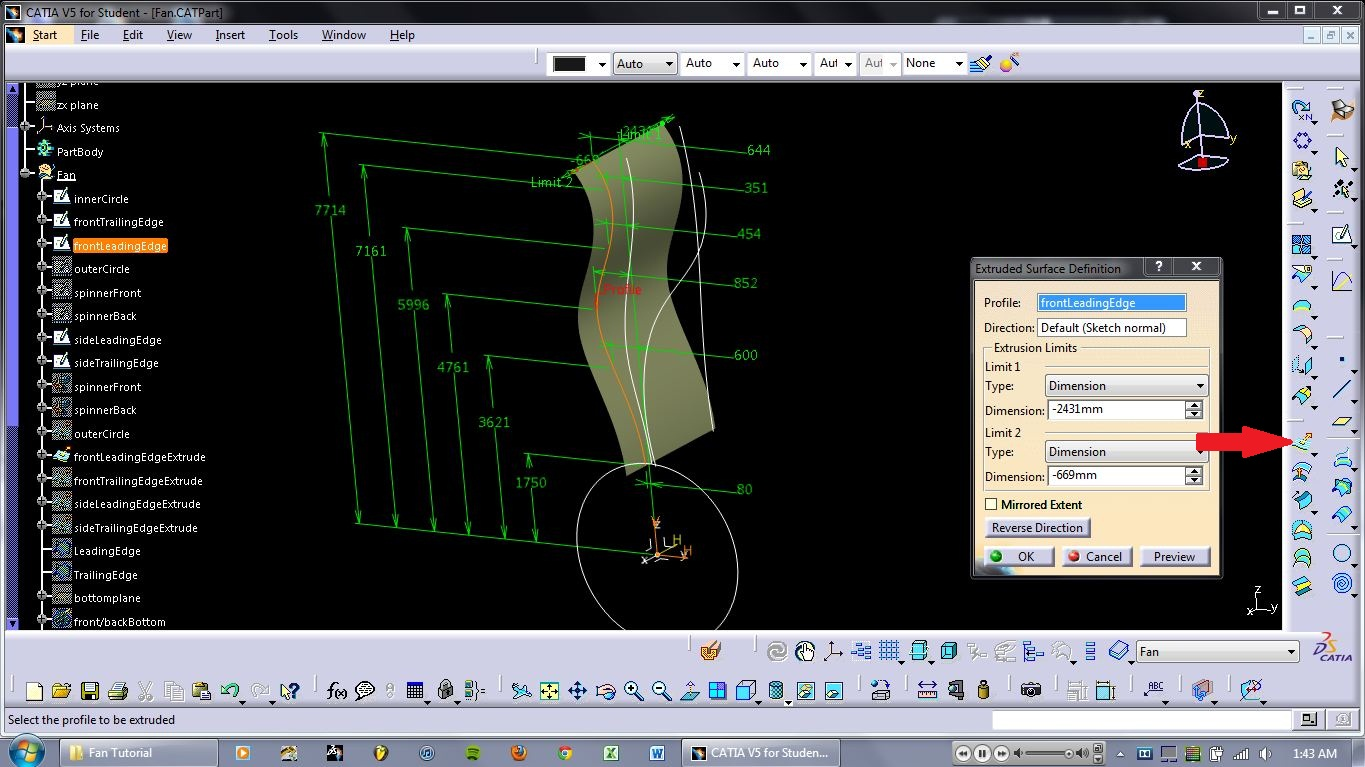7. ### Step 7:

7. Extrude the 2nd spline.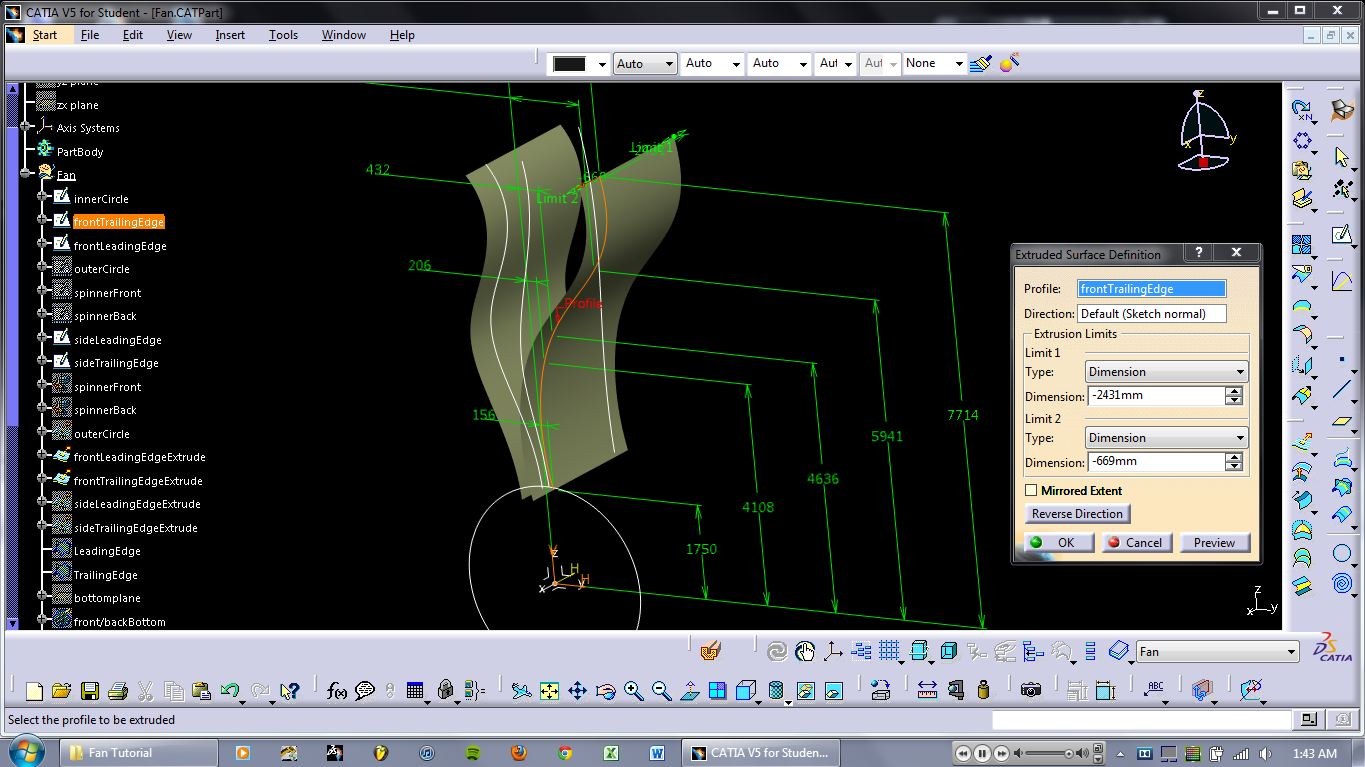8. ### Step 8:

8. and the 3rd...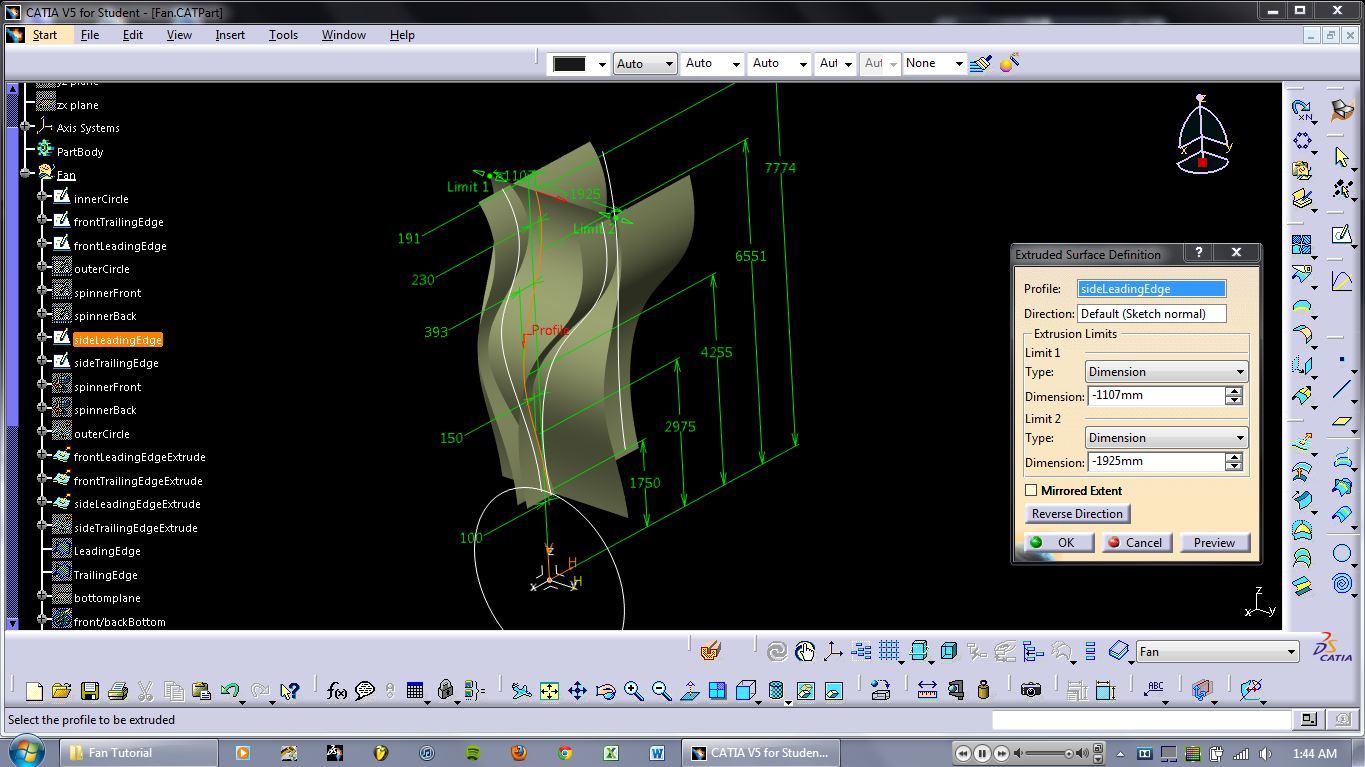9. ### Step 9:

9. and the 4th... You should end up with a kind-of box.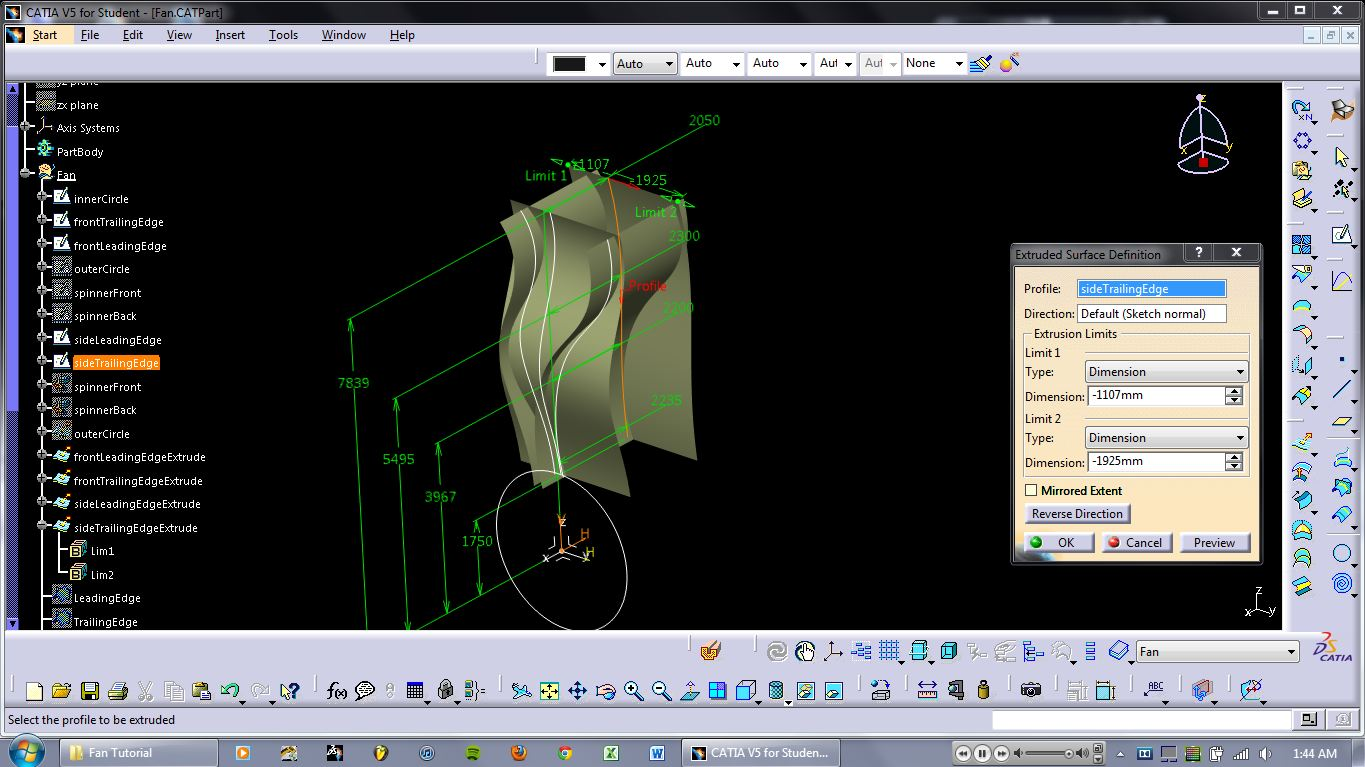10. ### Step 10:

10. Create an intersection curve as shown.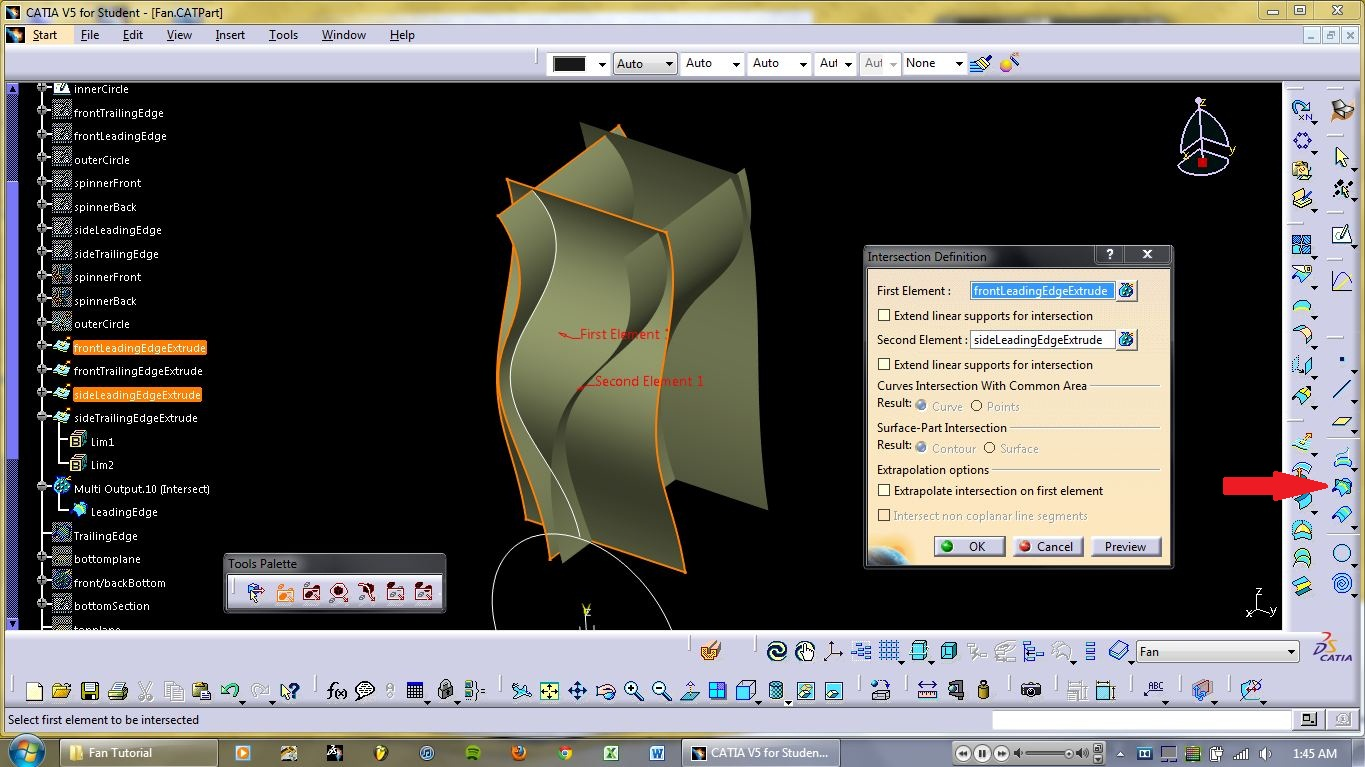11. ### Step 11:

11. Create another intersection curve.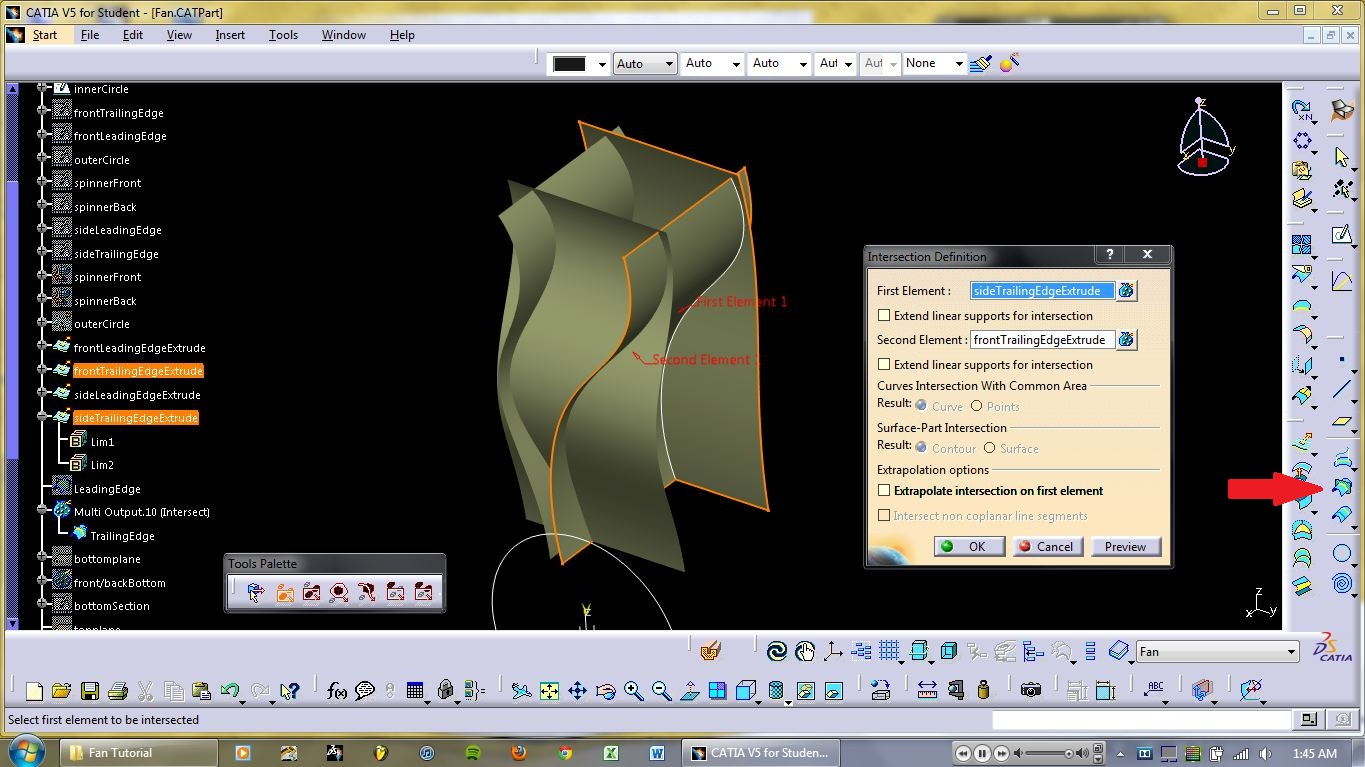12. ### Step 12:

12. Create the plane as shown.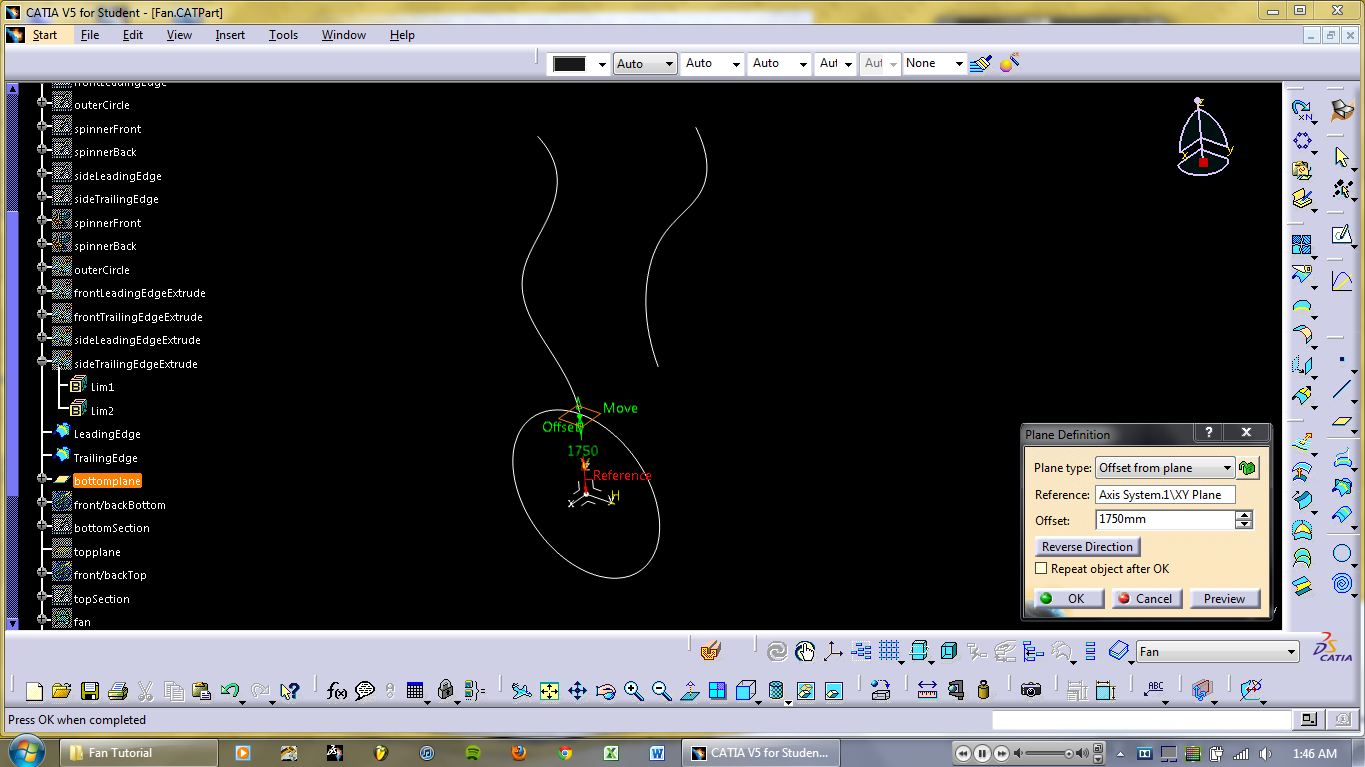13. ### Step 13:

13. Create 2 intersection points. Click the button shown to add the 2 curves.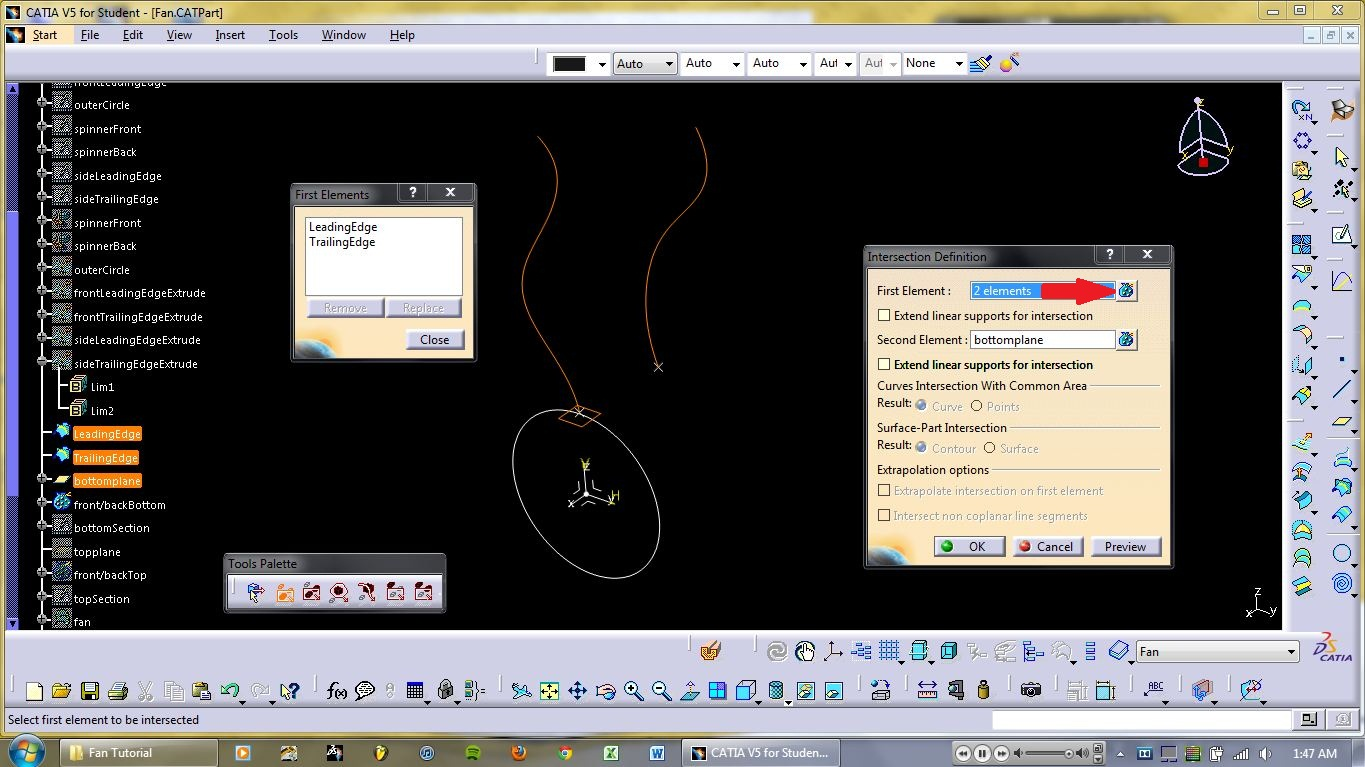14. ### Step 14:

14. Enter sketcher on the plane you just made and make the following shape. The two endpoints should be coincident with the two intersection points you just made.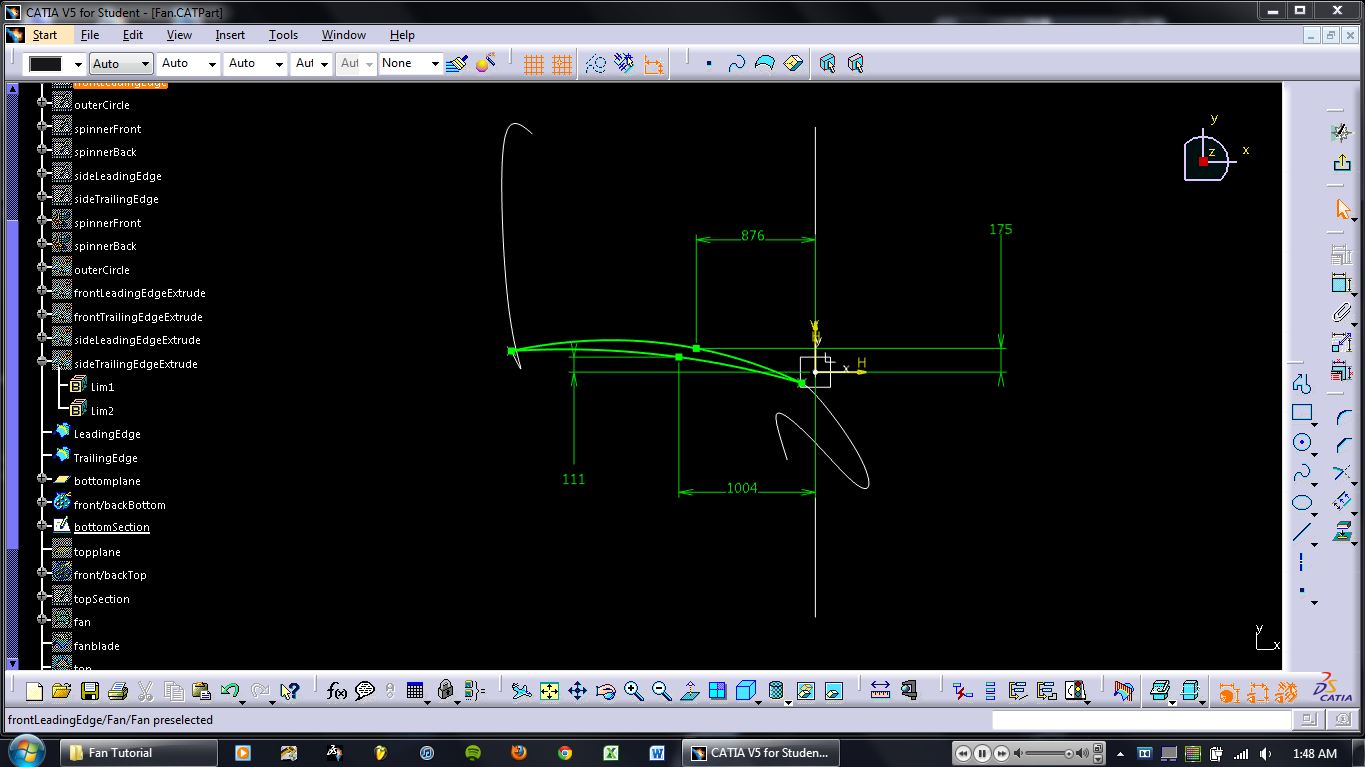15. ### Step 15:

15. Make another plane as shown.